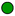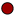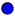Jr Math section of MathBitsNotebook.comSigned Numbers (Integers)NGMS-6NGMS-7NGMS-8

Integers (opposites, additive inverse, double negatives, order, info to know)Rounding Integers (includes rounding negative numbers)Signed Numbers as Opposites (list of applied examples of opposites)*
Absolute Value (definition, distance between points, computations)Graphing - Number Lines (integers, rationals, order)Graphing - Coordinate Axes (plotting, reflections, distance)Rational Numbers (definition, arithmetic procedures - numeric, conceptual, graphic)Arithmetic on Number Lines (add, subtract, multiply, divide, signed numbers)Using Counters with Integers (add, subtract, connection to subtract rule)*
Arithmetic of Signed Numbers ("rules" for add, subtract, multiply, divide)PRACTICE: • Practice with Signed Numbers• Applied Practice with Signed Numbers• Practice with Graphs and Number Lines• Practice with Absolute Value• Mixed Practice with Signed Numbers (Round 1, Round2 - basic skills)• Practice with Signed Numbers• Vocabulary - Word Guess Game (Jr level topical vocabulary/concept game)